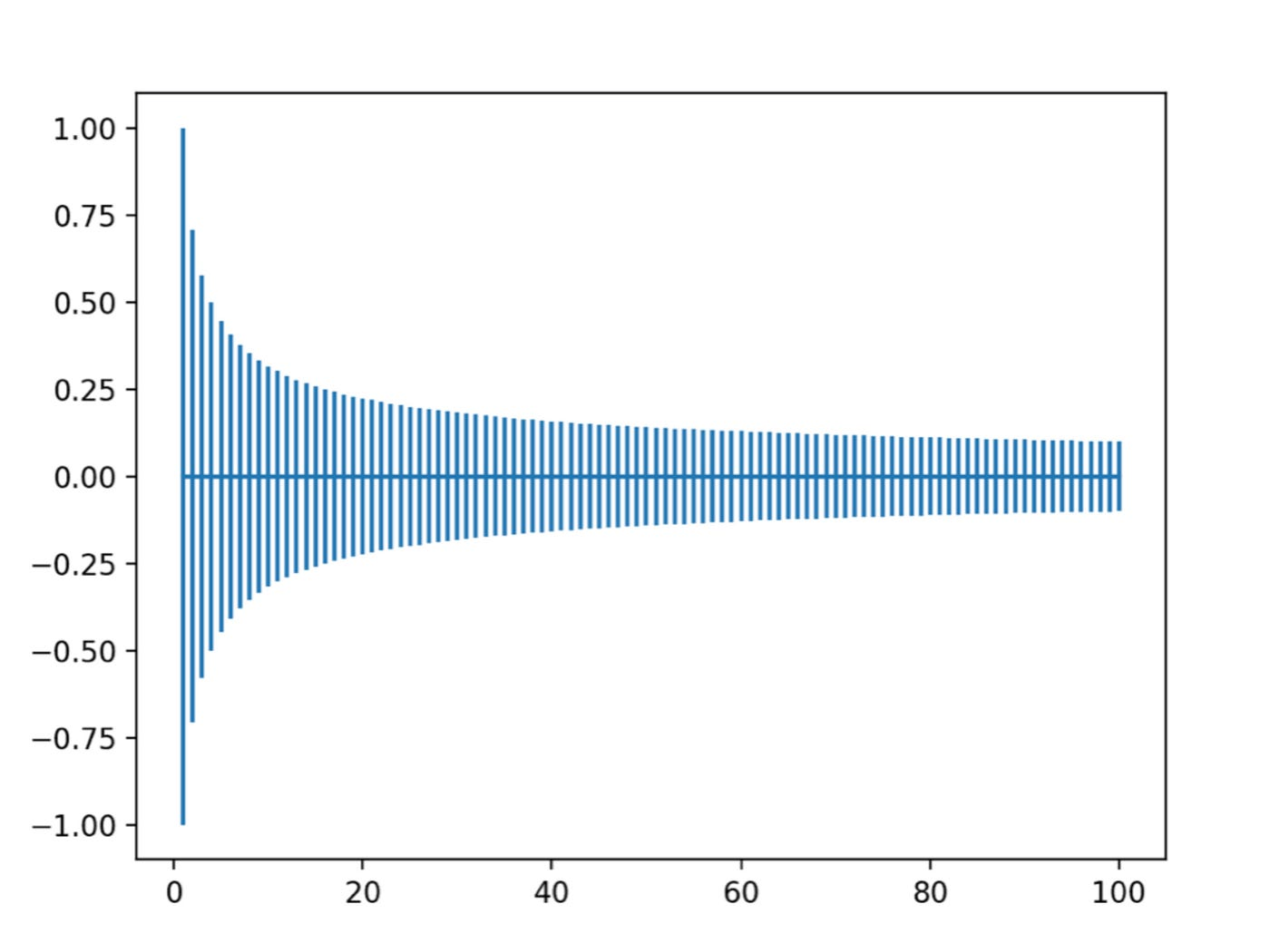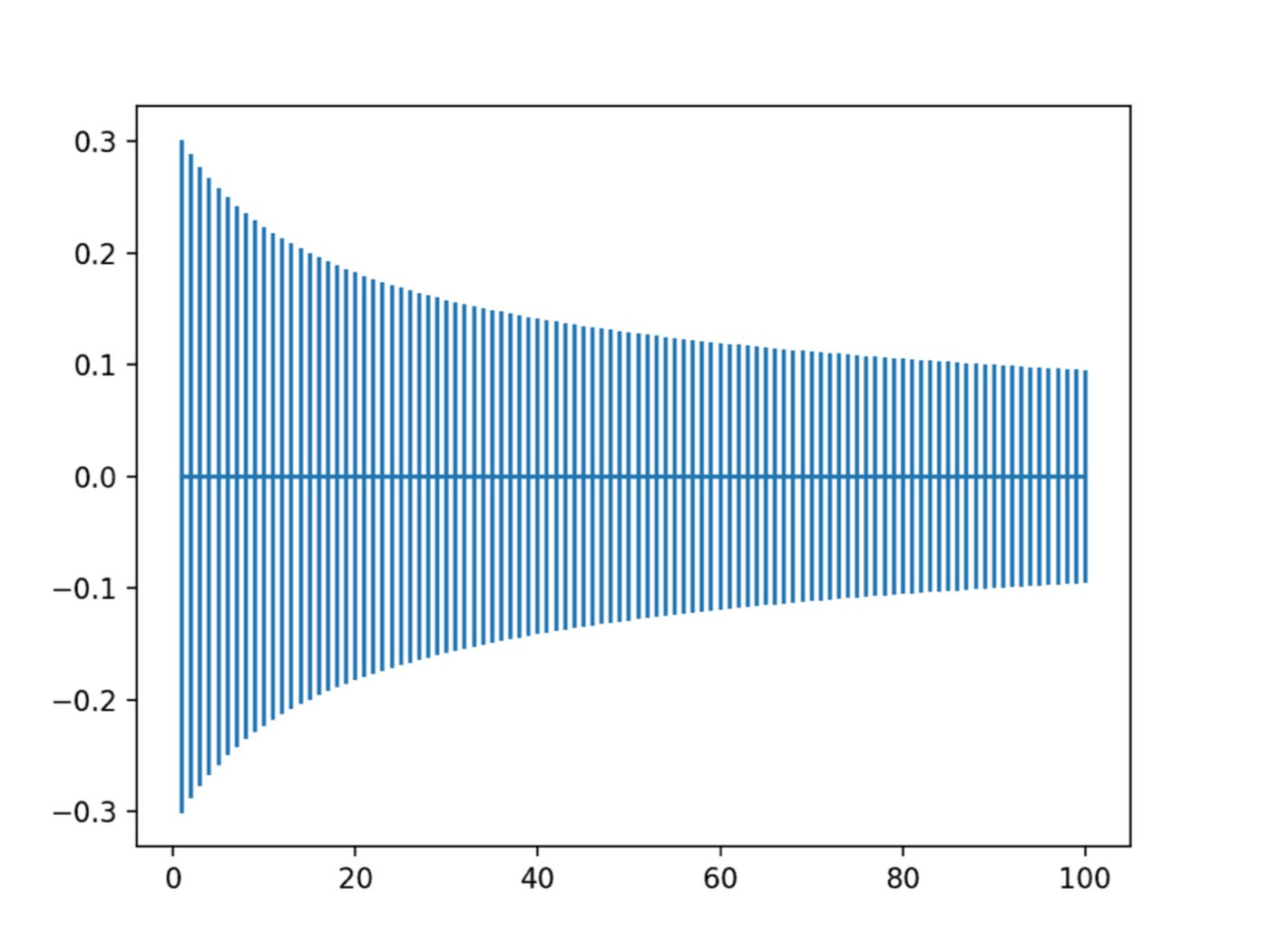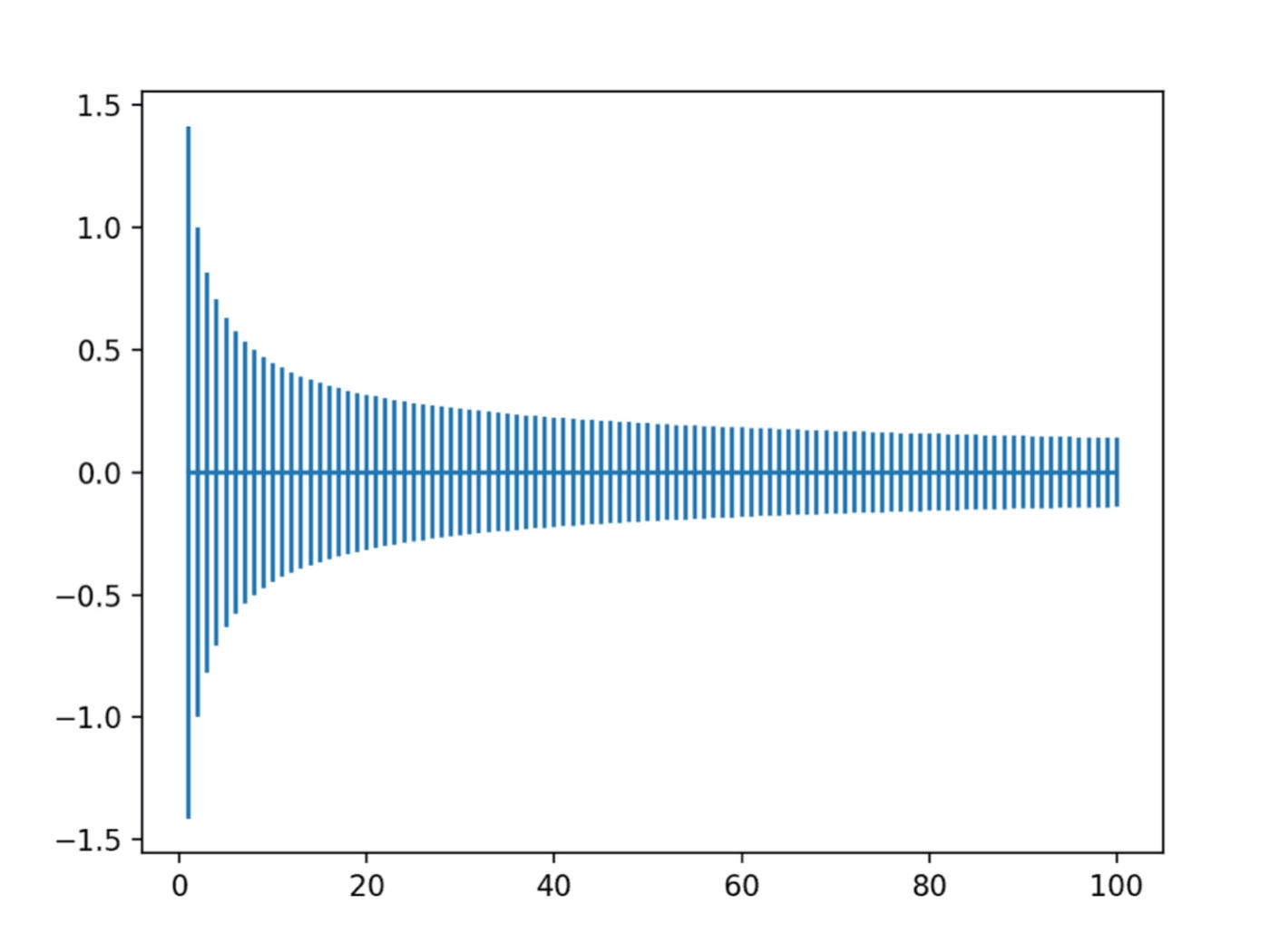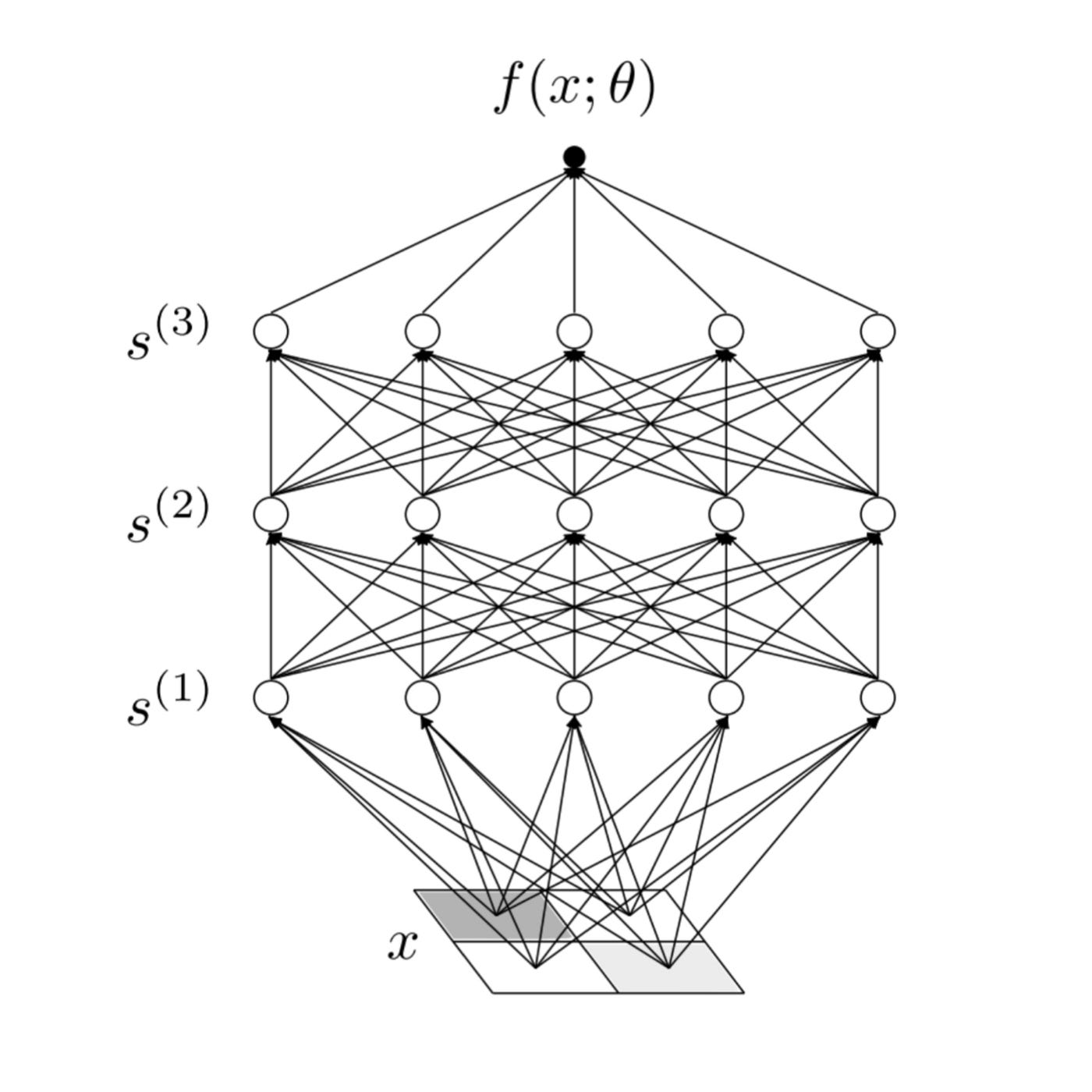# Initialization of Deep Learning

Thanks to substantial investments into computer technology, modern artificial intelligence(AI) systems can now come equipped with many billions of elementary components. When these components are properly initialized and then trained, AI can accomplish tasks once considered so incredibly complex that philosophers have previously argued that only natural intelligence systems — i.e. humans — could perform them. Behind much of this success in AI is deep learning. Deep learning uses artificial neural networks as an underlying model for AI: while loosely based on biological neural networks such as your brain, artificial neural networks are probably best thought of as an especially nice way of specifying a flexible set of functions, built out of many basic computational blocks called neurons. This model of computation is actually quite different from the one used to power the computer you’re likely using to read this book. In particular, rather than programming a specific set of instructions to solve a problem directly, deep learning models are trained on data from the real world and learn how to solve problems.

The real power of the deep learning framework comes from deep neural networks, with many neurons in parallel organized into sequential computational layers, learning useful representations of the world. Such representation learning transforms data into increasingly refined forms that are helpful for solving an underlying task, and is thought to be a hallmark of success in intelligence, both artificial and biological. Despite these successes and the intense interest they created, deep learning theory is still in its infancy. Indeed, there is a serious disconnect between theory and practice: while practitioners have reached amazing milestones, they have far outpaced the theorists, whose analyses often involve assumptions so unrealistic that they lead to conclusions that are irrelevant to understanding deep neural networks as they are typically used. More importantly, very little theoretical work directly confronts the deep of deep learning, despite a mass of empirical evidence for its importance in the success of the framework. The goal of this book is to put forth a set of principles that enable us to theoretically analyze deep neural networks of actual relevance. To initialize you to this task, in the rest of this chapter we’ll explain at a very high-level both(i)why such a goal is even attainable in theory and(ii)how we are able to get there in practice.

## Xavier Weight Initialization

The xavier initialization method is calculated as a random number with a uniform probability distribution (U) between the range -(1/sqrt(n)) and 1/sqrt(n), where n is the number of inputs to the node.

• weight = U [-(1/sqrt(n)), 1/sqrt(n)]

We can implement this directly in Python.

The example below assumes 10 inputs to a node, then calculates the lower and upper bounds of the range and calculates 1,000 initial weight values that could be used for the nodes in a layer or a network that uses the sigmoid or tanh activation function.

After calculating the weights, the lower and upper bounds are printed as are the min, max, mean, and standard deviation of the generated weights.

The complete example is listed below:

Running the example generates the weights and prints the summary statistics.

We can see that the bounds of the weight values are about -0.316 and 0.316. These bounds would become wider with fewer inputs and more narrow with more inputs.

We can see that the generated weights respect these bounds and that the mean weight value is close to zero with the standard deviation close to 0.17:

It can also help to see how the spread of the weights changes with the number of inputs.

For this, we can calculate the bounds on the weight initialization with different numbers of inputs from 1 to 100 and plot the result.

The complete example is listed below:

Running the example creates a plot that allows us to compare the range of weights with different numbers of input values.

We can see that with very few inputs, the range is large, such as between -1 and 1 or -0.7 to -7. We can then see that our range rapidly drops to about 20 weights to near -0.1 and 0.1, where it remains reasonably constant.Plot of Range of Xavier Weight Initialization With Inputs From One to One Hundred

The normalized xavier initialization method is calculated as a random number with a uniform probability distribution (U) between the range -(sqrt(6)/sqrt(n + m)) and sqrt(6)/sqrt(n + m), where n us the number of inputs to the node (e.g. number of nodes in the previous layer) and m is the number of outputs from the layer (e.g. number of nodes in the current layer).

• weight = U [-(sqrt(6)/sqrt(n + m)), sqrt(6)/sqrt(n + m)]

We can implement this directly in Python as we did in the previous section and summarize the statistical summary of 1,000 generated weights.

The complete example is listed below:

Running the example generates the weights and prints the summary statistics.

We can see that the bounds of the weight values are about -0.447 and 0.447. These bounds would become wider with fewer inputs and more narrow with more inputs.

We can see that the generated weights respect these bounds and that the mean weight value is close to zero with the standard deviation close to 0.17:

It can also help to see how the spread of the weights changes with the number of inputs.

For this, we can calculate the bounds on the weight initialization with different numbers of inputs from 1 to 100 and a fixed number of 10 outputs and plot the result.

The complete example is listed below:

Running the example creates a plot that allows us to compare the range of weights with different numbers of input values.

We can see that the range starts wide at about -0.3 to 0.3 with few inputs and reduces to about -0.1 to 0.1 as the number of inputs increases.

Compared to the non-normalized version in the previous section, the range is initially smaller, although transitions to the compact range at a similar rate.Plot of Range of Normalized Xavier Weight Initialization With Inputs From One to One Hundred

## Weight Initialization for ReLU

The “xavier” weight initialization was found to have problems when used to initialize networks that use the rectified linear (ReLU) activation function.

As such, a modified version of the approach was developed specifically for nodes and layers that use ReLU activation, popular in the hidden layers of most multilayer Perceptron and convolutional neural network models.

The current standard approach for initialization of the weights of neural network layers and nodes that use the rectified linear (ReLU) activation function is called “he” initialization.

It is named for Kaiming He, currently a research scientist at Facebook, and was described in the 2015 paper by Kaiming He, et al. titled “Delving Deep into Rectifiers: Surpassing Human-Level Performance on ImageNet Classification.”

## He Weight Initialization

The he initialization method is calculated as a random number with a Gaussian probability distribution (G) with a mean of 0.0 and a standard deviation of sqrt(2/n), where n is the number of inputs to the node.

• weight = G (0.0, sqrt(2/n))

We can implement this directly in Python.

The example below assumes 10 inputs to a node, then calculates the standard deviation of the Gaussian distribution and calculates 1,000 initial weight values that could be used for the nodes in a layer or a network that uses the ReLU activation function.

After calculating the weights, the calculated standard deviation is printed as are the min, max, mean, and standard deviation of the generated weights.

The complete example is listed below:

Running the example generates the weights and prints the summary statistics.

We can see that the bound of the calculated standard deviation of the weights is about 0.447. This standard deviation would become larger with fewer inputs and smaller with more inputs.

We can see that the range of the weights is about -1.573 to 1.433 which is close to the theoretical range of about -1.788 and 1.788, which is four times the standard deviation, capturing 99.7% of observations in the Gaussian distribution. We can also see that the mean and standard deviation of the generated weights are close to the prescribed 0.0 and 0.447 respectively:

It can also help to see how the spread of the weights changes with the number of inputs.

For this, we can calculate the bounds on the weight initialization with different numbers of inputs from 1 to 100 and plot the result.

The complete example is listed below:

Running the example creates a plot that allows us to compare the range of weights with different numbers of input values.

We can see that with very few inputs, the range is large, near -1.5 and 1.5 or -1.0 to -1.0. We can then see that our range rapidly drops to about 20 weights to near -0.1 and 0.1, where it remains reasonably constant.Plot of Range of He Weight Initialization With Inputs From One to One Hundred

## An Effective Theory Approach

While modern deep learning models are built up from seemingly innumerable elementary computational components, a first-principles microscopic description of how a trained neural network computes a function from these low-level components is entirely manifest. This microscopic description is just the set of instructions for transforming an input through the many layers of components into an output. Importantly, during the training process, these components become very finely-tuned, and knowledge of the particular tunings is necessary for a system to produce useful output.

Unfortunately, the complexity of these tunings obscures any first-principles macroscopic understanding of why a deep neural network computes a particular function and not another. With many neurons performing different tasks as part of such a computation, it seems hopeless to think that we can use theory to understand these models at all, and silly to believe that a small set of mathematical principles will be sufficient for that job.

Fortunately, theoretical physics has a long tradition of finding simple effective theories of complicated systems with a large number of components. The immense success of the program of physics in modelling our physical universe suggests that per-haps some of the same tools may be useful for theoretically understanding deep neural networks. To motivate this connection, let’s very briefly reflect on the successes of thermo-dynamics and statistical mechanics, physical theories that together explain from microscopic first principles the macroscopic behavior of systems with many elementary constituents.

A scientific consequence of the Industrial Age, thermo dynamics arose out of an effort to describe and innovate upon the steam engine — a system consisting of many many particles and perhaps the original black box. The laws of thermodynamics, derived from careful empirical observations, were used to codify the mechanics of steam, providing ahigh-level understanding of these macroscopic artificial machines that were transforming society. While the advent of thermodynamics led to tremendous improvements in the efficiency of steam power, its laws were in no way fundamental.

It wasn’t until much later that Maxwell, Boltzmann, and Gibbs provided the missing link between experimentally-derived effective description on the one hand and a first-principles theory on the other hand. Their statistical mechanics explains how the macroscopic laws of thermodynamics describing human-scale machines could arise statistically from the deterministic dynamics of many microscopic elementary constituents. From this perspective, the laws of thermodynamics were emergent phenomena that only appear from the collective statistical behavior of a very large number of microscopic particles. In fact, it was the detailed theoretical predictions derived from statistical mechanics that ultimately led to the general scientific acceptance that matter is really comprised of molecules and atoms. Relentless application of statistical mechanics led to the discovery of quantum mechanics, which is a precursor to the invention of the transistor that powers the Information Age, and — taking the long view — is what has allowed us to begin to realize artificial machines that can think intelligently.

Notably, these physical theories originated from a desire to understand artificial human-engineered objects, such as the steam engine. Despite a potential misconception, physics doesn’t make a distinction between natural and artificial phenomena. Most fundamentally, it’s concerned with providing a unified set of principles that account for past empirical observations and predict the result of future experiments; the point of theoretical calculations is to connect measurable outcomes or observables directly to the fundamental underlying constants or parameters that define the theory. This perspective also implies a trade-off between the predictive accuracy of a model and its mathematical tractability, and the former must take precedence over the latter for any theory to be successful: a short tether from theory to physical reality is essential. When successful, such theories provide a comprehensive understanding of phenomena and empower practical advances in technology, as exemplified by the statistical-physics bridge from the Age of Steam to the Age of Information.

For our study of deep learning, the key takeaway from this discussion is that a theoretical matter simplifies when it is made up of many elementary constituents. Moreover, unlike the molecules of water contained in a box of steam — with their existence once being a controversial conjecture in need of experimental verification — the neurons com-prising a deep neural network are put in (the box) by hand. Indeed, in this case weal ready understand the microscopic laws –how a network computes — and so instead our task is to understand the new types of regularity that appear at the macroscopic scale –why it computes one particular function rather than another — that emerge from the statistical properties of these gigantic deep learning models.Figure 1: A graph of a simple multilayer neural network, depicting how the input x is transformed through a sequence of intermediate signals, s(1),s(2), and s(3), into the out-put f(x;θ). The white circles represent the neurons, the black dot at the top represents the network output, and the parametersθare implicit; they weight the importance of the different arrows carrying the signals and bias the firing threshold of each neuron.

# The Theoretical Minimum

The method is more important than the discovery, because the correct method of research will lead to new, even more valuable discoveries. Lev Landau .In this section, we’ll give a high-level overview of our method, providing a minimal explanation for why we should expect a first-principles theoretical understanding of deep neural networks to be possible. We’ll then fill in all the details in the coming chapters. In essence, a neural network is a recipe for computing a function built out of many computational units called neurons. Each neuron is itself a very simple function that considers a weighted sum of incoming signals and then fires in a characteristic way by comparing the value of that sum against some threshold. Neurons are then organized in parallel into layers, and deep neural networks are those composed of multiple layers in sequence. The network is parametrized by the firing thresholds and the weighted connections between the neurons, and, to give a sense of the potential scale, current state-of-the-art neural networks can have over 100 billion parameters. A graph depicting the structure of a much more reasonably-sized neural network is shown in Figure 1.For a moment, let’s ignore all that structure and simply think of a neural network as a parameterized function

From this, we see that to really describe the properties of multilayer neural networks, i.e. to understand deep learning, we need to study large-but-finite-width networks. In this way, we’ll be able to find a macroscopic effective theory description of realistic deep neural networks.

--

--